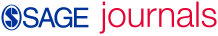# Finding globally optimal macrostructure in multiple relation, mixed-mode social networks

Posted on 2020-10-22 - 12:06

From the outset, computational sociologists have stressed leveraging multiple relations when blockmodeling social networks. Despite this emphasis, the majority of published research over the past 40 years has focused on solving blockmodels for a single relation. When multiple relations exist, a reductionist approach is often employed, where the relations are stacked or aggregated into a single matrix, allowing the researcher to apply single relation, often heuristic, blockmodeling techniques. Accordingly, in this article, we develop an exact procedure for the exploratory blockmodeling of multiple relation, mixed-mode networks. In particular, given (a) ${N}_{1}$ actors, (b) ${N}_{2}$ events, (c) an $\left({N}_{1}×{N}_{1}\right)$ binary one-mode network depicting the ties between actors, and (d) an $\left({N}_{1}×{N}_{2}\right)$ binary two-mode network representing the ties between actors and events, we use integer programming to find globally optimal $\left({P}_{1}×{P}_{1}|{P}_{1}×{P}_{2}\right)$ image matrices and partitions, where ${P}_{1}$ and ${P}_{2}$ represent the number of actor and event positions, respectively. Given the problem’s computational complexity, we also develop an algorithm to generate a minimal set of non-isomorphic image matrices, as well as a complementary, easily accessible heuristic using the network analysis software Pajek. We illustrate these concepts using a simple, hypothetical example, and we apply our techniques to a terrorist network.

## CITE THIS COLLECTION

or
Select your citation style and then place your mouse over the citation text to select it.

## Usage metrics## AUTHORS (3)Matthew F DabkowskiNeng FanRonald Breiger

## CATEGORIES

figshare. credit for all your research.